# Linear Programming Class 12 Mathematics Important Questions## myCBSEguide App

CBSE, NCERT, JEE Main, NEET-UG, NDA, Exam Papers, Question Bank, NCERT Solutions, Exemplars, Revision Notes, Free Videos, MCQ Tests & more.

Linear Programming Class 12 Mathematics Important Questions. myCBSEguide has just released Chapter Wise Question Answers for class 12 Maths. There chapter wise Practice Questions with complete solutions are available for download in myCBSEguide website and mobile app. These Questions with solution are prepared by our team of expert teachers who are teaching grade in CBSE schools for years. There are around 4-5 set of solved Chapter 12 Linear Programming Mathematics Extra Questions from each and every chapter. The students will not miss any concept in these Chapter wise question that are specially designed to tackle Board Exam. We have taken care of every single concept given in CBSE Class 12 Mathematics syllabus and questions are framed as per the latest marking scheme and blue print issued by CBSE for class 12.

Class 12 Chapter 12 Maths Extra Questions

## Class 12 Linear Programming Mathematics Extra Questions

Chapter 12 Linear Programming

1. Maximise Z = 3x + 4y subject to the constraints: x + y ≤ 4, x ≥ 0, y ≥ 0.
1. Maximum Z = 16 at (0, 4)
2. Maximum Z = 19 at (1, 5)
3. Maximum Z = 18 at (1, 4)
4. Maximum Z = 17 at (0, 5)
2. A linear programming problem is one that is concerned with
1. finding the optimal value (maximum or minimum) of a linear function of several variables
2. finding the limiting values of a linear function of several variables
3. finding the lower limit of a linear function of several variables
4. finding the upper limits of a linear function of several variables
3. Maximise Z = x + y subject to x + 4y ≤ 8, 2x + 3y ≤ 12, 3x + y ≤ 9, x ≥ 0, y ≥ 0.
1. {tex}2\frac{{10}}{{11}}{/tex}
2. {tex}3\frac{{19}}{{31}}{/tex}
3. {tex}3\frac{{10}}{{11}}{/tex}
4. {tex}3\frac{9}{{11}}{/tex}
4. A farmer mixes two brands P and Q of cattle feed. Brand P, costing Rs 250 per bag, contains 3 units of nutritional element A, 2.5 units of element B and 2 units of element C. Brand Q costing Rs 200 per bag contains 1.5 units of nutritional element A, 11.25 units of element B, and 3 units of element C. The minimum requirements of nutrients A, B and C are 18 units, 45 units and 24 units respectively. Determine the number of bags of each brand which should be mixed in order to produce a mixture having a minimum cost per bag? What is the minimum cost of the mixture per bag?
1. 5 bags of brand P and 6 bags of brand Q; Minimum cost of the mixture = Rs 2250
2. 3 bags of brand P and 6 bags of brand Q; Minimum cost of the mixture = Rs 1950
3. 6 bags of brand P and 6 bags of brand Q; Minimum cost of the mixture = Rs 2350
4. 4 bags of brand P and 6 bags of brand Q; Minimum cost of the mixture = Rs 2150
5. The feasible solution for an LPP is shown in Figure. Let Z = 3x – 4y be the objective function. Minimum of Z occurs at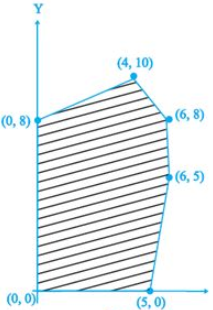1. (0, 8)
2. (0, 0)
3. (5, 0)
4. (4, 10)
6. A corner point of a feasible region is a point in the region which is the ________ of two boundary lines.
7. In a L.P.P, the linear inequalities or restrictions on the variables are called ________.
8. In a LPP if the objective function Z = ax + by has the same maximum value on two corner points of the feasible region, then every point on the line segment joining these two points give the same ________ value.
9. A small firm manufactures necklaces and bracelets. The total number of necklaces and bracelets that it can handle per day is at most 24. It takes one hour to make a bracelet and half an hour to make a necklace. The maximum number of hours available per day is 16. If the profit on a necklace is Rs 100 and that on a bracelet is Rs 300. Formulate on L.P.P. for finding how many of each should be produced daily to maximise the profit? It is being given that at least one of each must be produced.
10. In order to supplement daily diet, a person wishes to take some X and some wishes Y tablets. The contents of iron, calcium and vitamins in X and Y (in milligram per tablet) are given as below:

 Tablets Iron Calcium Vitamin X 6 3 2 Y 2 3 4

The person needs at least 18 milligram of iron, 21 milligram of calcium and 16 milligram of vitamins. The price of each tablet of X and Y is Rs 2 and Re 1 respectively. How many tablets of each should the person take in order to satisfy the above requirement at the minimum cost?

11. A factory owner purchases two types of machine A and B for his factory. The requirements and the limitations for the machines are as follows,

MachineArea OccupiedLabour forceDaily
on each machineoutput (in units)
A1000 m212 men60
B1200 m28 men40

He has maximum area 9000 m2 available and 72 skilled labours who can operate both the machines. How many machines of each type should he buy to maximize the daily out put?

12. Determine the minimum value of Z = 3x + 2y (if any), if the feasible region for an LPP is shown in Fig.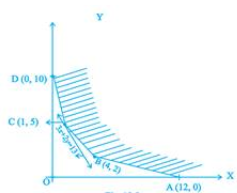13. Minimise Z = 13x – 15y, subject to the constraints: {tex}x + y \leq 7,2 x – 3 y + 6 \geq 0 , x \geq 0 , y \geq 0{/tex}.
14. A manufacturer of electronic circuits has a stock of 200 resistors, 120 transistors and 150 capacitors and is required to produce two types of circuits A and B. Type A requires 20 resistors, 10 transistors and 10 capacitors. Type B requires 10 resistors, 20 transistors and 30 capacitors. If the profit on type A circuit is Rs 50 and that on type B circuit is Rs 60, formulate this problem as a LPP so that the manufacturer can maximise his profit.
15. The feasible region for a LPP is shown in Fig. 12.10. Evaluate Z = 4x + y at each of the corner points of this region. Find the minimum value of Z, if it exists.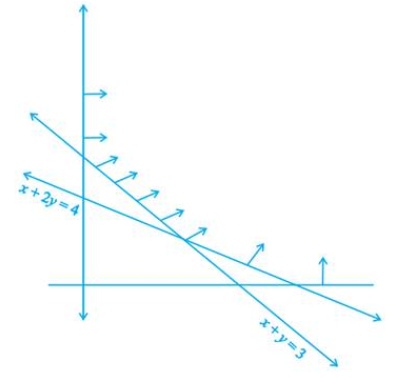16. There are two types of fertilizers F1 and F2. F1 consists of 10% nitrogen and 6% phosphoric acid and F2 consists of 5% nitrogen and 10% phosphoric acid. After testing the soil conditions, a farmer finds that she needs atleast 14kg of nitrogen and 14 kg of phosphoric acid for her crop. If F1 costs Rs 6/kg and F2 costs Rs 5/kg, determine requirements are met at a minimum cost. What is the minimum cost?
17. A factory makes tennis rackets and cricket bats. A tennis racket takes 1.5 hours of machine time and 3 hours of craftman’s time in its making while a cricket bat takes 3 hour of machine time an 1 hour of craftman’s time. In a day, the factory has the availability of not more than 42 hours of machine time and 24 hours of craftsman’s time.

1. What number of rackets and bats must be made if the factory is t work at full capacity?
2. If the profit on a racket and on a bat is Rs 20 and Rs 10 respectively, find the maximum profit of the factory when it works at full capacity.
18. Two tailors A and B earn Rs. 150 and Rs. 200 per day, respectively. A can stitch 6 shirts and 4 pants per day, while B can stitch 10 shirts and 4 pants per day. How many days shall each work, if it is desired to produce at least 60 shirts and 32 pants at a minimum labour cost? Make it as an LPP and solve the problem graphically.

Chapter 12 Linear Programming

Solution

1. Maximum Z = 16 at (0, 4)
Explanation: Objective function is Z = 3x + 4 y ……(1).
The given constraints are : x + y ≤ 4, x ≥ 0, y ≥ 0.
The corner points obtained by constructing the line x+ y= 4, are (0,0),(0,4) and (4,0).

 Corner points Z = 3x +4y O ( 0 ,0 ) Z = 3(0)+4(0) = 0 A ( 4 , 0 ) Z = 3(4) + 4 (0) = 12 B ( 0 , 4 ) Z = 3(0) + 4 ( 4) = 16 …( Max. )

therefore Z = 16 is maximum at ( 0 , 4 ).

1. finding the optimal value (maximum or minimum) of a linear function of several variables
Explanation: A linear programming problem is one that is concerned with finding the optimal value (maximum or minimum) of a linear function of several variables.
1. {tex}3\frac{{10}}{{11}}{/tex}
Explanation: Here , Maximise Z = x + y subject to x + 4y ≤ 8, 2x + 3y ≤ 12, 3x + y ≤ 9, x ≥ 0, y ≥ 0.

 Corner points Z = x + y P( 0 , 0 ) 0 Q(3 , 0) 3 R( 0, 2 ) 2 S(28/11 , 15/11 ) 43/11.(Max.)

Hence the maximum value is 43/11 = 3{tex}10\over11{/tex}

1. 3 bags of brand P and 6 bags of brand Q; Minimum cost of the mixture = Rs 1950
Explanation: Let number of bags of cattle feed of brand P = x
And number of bags of cattle feed of brand Q = y
Therefore , the above L.P.P. is given as :
Minimise, Z = 250x +200y , subject to the constraints : 3 x + 1.5y ≥ 80, 2.5x + 11.25y ≥ 45, 2x + 3y ≥ 24 , x,y ≥ 0.,

 Corner points Z =250 x +200 y C( 0,12 ) 2400 B (18,0) 4500 D(3,6 ) 1950 (Min.) A(9,2) 2650

Here Z = 1950 is minimum.
i.e. 3 bags of brand P and 6 bags of brand Q; Minimum cost of the mixture = Rs 1950.

1. (0, 8)
Explanation:

 Corner points Z = 3x – 4y (0, 0) 0 (5,0) 15 (6,8) -14 (6 ,5) -2 (4,10) -28 (0,8) -32……………..(Min.)

The minimum value occurs at (0,8)

1. Intersection
2. Linear constraints
3. maximum
4. Let number of necklaces and bracelets produced by firm per day be x and y, respectively.Given that the maximum number of both necklaces and bracelets that firm can handle per day is almost 24.
which means that the firm can produce maximum 24 items which includes both necklaces and bracelets per day. Hence the inequality related to the number constraint is given as
{tex}\therefore{/tex} x + y {tex}\leq{/tex} 24
Also given that It takes one hour per day to make a bracelet and half an hour per day to make a necklace and maximum number of hours available per day is 16.
Hence the inequality representing the hour constraint is given as
{tex}\therefore{/tex} x + {tex}\frac 12{/tex}y {tex}\leq{/tex} 16 {tex}\Rightarrow{/tex} ( when multiplying throughout the inequality by 2 we get )
2x + y {tex}\leq{/tex} 32
Also the non negative constraints which restricts the feasible region of the problem within the first quadrant is given as x{tex}\geq{/tex}0, y{tex}\geq{/tex}0, since the given situations are real world connected and cannot have the solution as negative which means that the values of the variables x and y are non negative.
Let z be the objective function which represents the total maximum profit.Hence the equation of the profit function Z is given as
{tex}\therefore{/tex} z = 100x + 300y, which is to be maximised
subject to the constraints,
x + y {tex}\leq{/tex} 24
2x + y {tex}\leq{/tex}32
x, y {tex}\geq{/tex}0
5. Let the person takes x units of tablet X and y unit of tablet Y.
So, from the given information, we have
{tex}6x + 2y \geqslant 18 \Rightarrow 3x + y \geqslant 9\,…(i){/tex}
{tex}3x + 3y \geqslant 21 \Rightarrow x + y \geqslant 7\,…(ii){/tex}
And {tex}2x + 4y \geqslant 16 \Rightarrow x + 2y \geqslant 8\,\,\,…(iii){/tex}
Also, we know that here, {tex}x \geqslant 0,y \geqslant 0\,…(iv){/tex}
The price of each tablet of X and Y is Rs 2 and Rs 1, respectively.
So, the corresponding LPP is minimise Z = 2x + y subject to {tex}3x + y \geqslant 9,x + y \geqslant 7x,x + y \geqslant 7,{/tex} {tex}x + 2y \geqslant 8,x \geqslant 0,y \geqslant 0{/tex}
From the shaded graph, we see that for the shown unbounded region, we have coordinates of corner points A, B, C and D as (8,0),(6,1),(1,6) and (0,9) respectively.
[On solving x + 2y = 8 and x + y = 7 we get x = 6, y = 1 and on solving 3x + y = 9 and x + y = 7, we get x = 1, y = 6]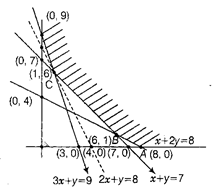Corner Points Values of Z = 2x + y (8, 0) 16 (6, 1) 13 (1, 6) 8 (minimum) (0, 9) 9

Thus, we see that 8 is the minimum value of Z at the corner point (1, 6). Here we see that the feasible region is unbounded. Therefore, 8 may or may not be the minimum value of Z. To decide this issue, we graph the inequality
2x + y < 8 …(v)
And check whether the resulting open half has points in common with feasible region or not. If it has common point, then 8 will not be the minimum value of Z, otherwise 8 will be the minimum value of Z.
Thus, from the graph it is clear that, it has no common point.
Therefore, Z = 2x + y has 8 as minimum value subject to the given constraint.
Hence, the person should take 1 unit of X tablet and 6 unit of Y tablets to satisfy the given requirements and at the minimum cost of Rs 8.

6. Let x machines of type A and y machines of type B be bought and let Z be the daily output.
{tex}\Rightarrow{/tex} {tex}Z = 60x + 40y{/tex} … (i)
Maximum area available = 9000 m2
{tex}\Rightarrow 1000 x + 1200 y \leq 9000{/tex} … (ii)
or
{tex}5 x + 6 y \leq 45{/tex}
Maximum labour available = 72 men
{tex}\Rightarrow 12 x + 8 y \leq 72{/tex}
{tex}3x + 2y < 18 {/tex}… (iii) Now, the mathematical formulation of given L.P.P. is as follows Maximize{tex} Z = 60x + 40y{/tex} Subject to constraints {tex}5x + 6y < 45{/tex}
{tex}3x + 2y < 18{/tex}
s.t. {tex}x > 0, y >0{/tex}
We draw the line {tex} 5x + 6y = 45{/tex} and {tex}3x + 2y = 18{/tex} and shaded the feasible region w.r.t. the constraint sign.
We observed that the feasible region is bounded and corner points are {tex}O(0, 0), A(6, 0){/tex}
{tex}\mathrm { B } \left( \frac { 9 } { 4 } , \frac { 45 } { 8 } \right){/tex} and {tex}\mathrm { C } \left( 0 , \frac { 15 } { 2 } \right){/tex}
Evaluating the Z at each corner point, we have

Corner PointsZ = 60x + 40y
{tex}O(0, 0){/tex}{tex}Z = 0{/tex}
{tex}A(6, 0){/tex}{tex}Z = 360{/tex} Maximum
{tex}\mathrm { B } \left( \frac { 9 } { 4 } , \frac { 45 } { 8 } \right){/tex}{tex}Z = 360{/tex} Maximum
{tex}\mathrm { C } \left( 0 , \frac { 15 } { 2 } \right){/tex}{tex}Z = 300{/tex}

Hence, either 6 machine of type A and no machine of type B or 2 machine of type A and 6 machine of type B be used to have maximum output.

7. The feasible region (R) is unbounded. Therefore, a minimum of Z may or may not exist. If it exists, it will be at the corner point Fig.
Corner PointValue of Z
A (12, 0)3(12) + 2(0) = 36
B(4, 2)3(4) + 2(2) = 16
C(1, 5)3(1) + 2(5) = 13 (smallest)
D(0, 10)3(0) + 2(10) = 20Let us graph 3x + 2y < 13. We see that the open half plane determined by 3x + 2y < 13 and R do not have a common point. So, the smallest value 13 is the minimum value of Z.

8. Consider{tex} x + y = 7{/tex}
When {tex}x = 0,\ then\ y = 7{/tex} and
when {tex}y = 0,\ then\ x = 7{/tex}
So, A(0, 7) and B(7, 0) are the points on line
{tex}x + y = 7{/tex}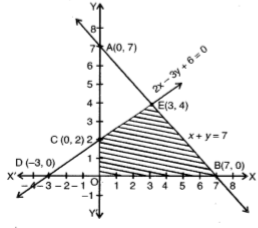Consider {tex}2x – 3y + 6 = 0{/tex}
When x = 0, then y = 2 and when y = 0, then x = – 3, So C(0, 2) and D(-3, 0) are the points on line {tex}2x – 3y + 6 = 0{/tex}
Also, we have x > 0 and v > 0.
The feasible region OBEC is bounded, so, minimum value will obtain at a comer point of this feasible region.
Corner points are O(0, 0), B(7, 0), E(3, 4) and C(0, 2)
{tex}Z = 13x – 15y {/tex}
At {tex}O(0, 0), Z = 0{/tex}
At {tex}B(7, 0), Z = 13(7) – 15(0) = 91 {/tex}
At {tex}E(3, 4), Z = 13(3) – 15(4) = -21 {/tex}
At {tex}C(0, 2), Z = 13(0) – 15(2){/tex}
= -30 (minimum)
Hence , the minimum value is -30 at the point (0, 2).
9. Let the manufacturer produce x units of type A circuit and y units of type B circuits.
From the given information, we have following corresponding constraint table.

Type A(x)Type B(y)Maximum stock
Resistors2010200
Transistors1020120
Capacitors1030150
ProfitRs 50Rs 60

Thus, we see that total profit Z = 50x + 60y (in Rs).
Now, we have the following mathematical model for the given problem.
Maximise Z = 50x + 60y ….. (i)
Subject to the constraints.
{tex}20x + 10y \leqslant 200{/tex} [resistor constraint] {tex} \Rightarrow 2x + y \leqslant 20{/tex}…… (ii)
And {tex}10x + 20y \leqslant 20{/tex} [transistor constraint] {tex} \Rightarrow x + 2y \leqslant 2{/tex} …. (iii)
And {tex}10x + 30y \leqslant 50{/tex} [capacitor constraint] {tex} \Rightarrow x + 3y \leqslant 5{/tex}….. (iv)
And {tex}x \leqslant 0,y \leqslant 0{/tex} …. (v) {tex}x \geqslant 0,y \geqslant 0{/tex} ….{tex}(\upsilon ){/tex} [non-negative constraint] So, Maximise Z = 50x + 60y, subject to {tex}2x + y \leqslant 20,x + 2y \leqslant 12,x + 3y \leqslant 15,{/tex}{tex}x\ge0,y\ge0{/tex}

10. From the shaded region, it is clear that feasible region is unbounded with the corner points A(4, 0), B(2, 1) and C(0, 3).
Also, we have Z = 4x + y.
[Since, x + 2y = 4 and x + y = 3 {tex} \Rightarrow {/tex} y = 1 and x = 2]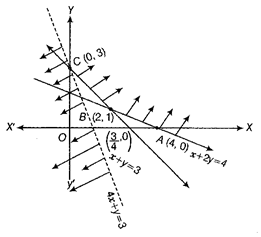Corner PointsCorresponding value of Z
(4, 0)16
(2, 1)9
(0, 3)3 (minimum)

Now, we see that 3 is the smallest value of Z at the corner point (0, 3). Note that here we see that the region is unbounded, therefore 3 may or may not be the minimum value of Z.
To decide this issue, we graph the inequality 4x + y < 3 and check whether the resulting open half plan has no point in common with feasible region otherwise, Z has no minimum value.
From the shown graph above, it is clear that there is no point in common with feasible region and hence Z has minimum value of 3 at (0, 3).

11.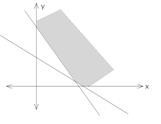Let the farmer use x Kg of F1 and y Kg of F2.
Z = 6x + 5y
{tex}\frac{{10x}}{{100}} + \frac{{5y}}{{100}} \geqslant 14{/tex}
{tex}\frac{{6x}}{{100}} + \frac{{10y}}{{100}} \geqslant 14{/tex}
{tex}x \geqslant 0,y \geqslant 0{/tex}
On solving these equations we get x = 100 and y = 80
Minimum cost
{tex}z = 6 \times 100 + 5 \times 80 = 600 + 400 = 1000{/tex}
Thus, minimum cost = Rs 1000.
Hence, 100 kg of fertilizer F1 and 80 kg of fertilizer F2 should be used so that nutrient requirements are met at minimum cost of Rs 1000.
12. Let the number of Tenis rackets and the number of cricket bats to be made in a day be x and y respectively.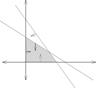Z = x + y
and also P = 20x + 10y
{tex}\frac{3}{2}x + 3y \leqslant 42{/tex}
{tex} \Rightarrow x + 2y \leqslant 28{/tex}
{tex}3x + y \leqslant 24{/tex}
{tex}x \geqslant 0,y \geqslant 0{/tex}
Solving ,x + 2y = 28 and 3x + y = 24,we get,x = 4,y = 12

1. Maximum Z = 16 at x = 4 ,y = 12
2. {tex}P = 20 \times 4 + 10 \times 12{/tex}
= 200
13. Let tailors A and B work for x and y days, respectively.
The given data can be written in tabular form as follows:

 Tailor A Tailor B Required Shirts per day 6 10 at least 60 Pants per day 4 4 at least 32 Wages per day 150 200

{tex}\therefore{/tex} The required linear programming problem is to minimize the wages per day. Let Z represent the objective function which represent the sum of the wages.Hence the equation of the objective function is given as (Z) = 150x + 200y
Subject to constraints
{tex}6 x + 10 y \geq 60{/tex} ( constraints for tailor A) ( dividing throughout by 2 we get)
{tex}\Rightarrow \quad 3 x + 5 y \geq 30{/tex}
{tex}4 x + 4 y \geq 32{/tex} ( constraints for tailor B) ( dividing throughout by 4 we get)
{tex}\Rightarrow \quad x + y \geq 8{/tex}
and {tex}x \geq 0 , y \geq 0{/tex} ( non negative constraints ,which will restrict the solution of the given inequalities in the first quadrant only)
On considering the inequalities as equations, we get
3x + 5y = 50 …(i)
x + y = 8 …(ii)
Table for line 3x + 5y = 30 is

 x 0 10 y 6 0

So, it passes through the points with coordinates (0, 6) and (10, 0).
On replacing the coordinates of the origin O (0, 0) is,{tex}3 x + 5 y \geq 30{/tex} we get
{tex}0 \geq 30{/tex} [which is false)
So, the half plane for the inequality of the line ( i) is away from the origin, which means that the origin is not a point in the feasible region.
Again, table for line ( ii) x + y = 8 is given below.

 x 0 8 y 8 0

So, it passes through the points with coordinates (0, 8) and (8, 0).
On replacing the origin O (0, 0) in {tex}x + y \geq 8{/tex} , we get
{tex}0 \geq 8{/tex} (which is false)
So, the half plane for the inequation of the line ( ii) is away from origin, which means that the point O( 0,0) is not a point in the feasible region of the inequality of the line (ii).
On solving Eqs. (i) and (ii), we get
x = 5 and y = 3
so, the point of intersection is P(5, 3).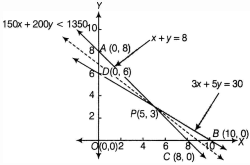from the above graph, APB is the feasible region and it is unbounded. The corner points are A(0, 8), P(5, 3) and B(10, 0).
The values of Z at corner points are as follows:

 Corner points Value of Z = 150x + 200y A(0, 8) Z = 150(0) + 200(8) = 1600 P(5, 3) Z = 150(5) + 200(3) = 1350 (minimum) B(10, 0) Z = 150(10) + 200(0) = 1500

from table, the minimum value of Z is 1350 at P( 5, 3).
As the feasible region is unbounded, therefore 1350 may or may not be the minimum value of Z. For this, we draw a graph of the inequality l50x + 200y < 1350 with dotted lines whose feasible region is towards the origin. Hence the feasible region has no point common with the feasible region of the inequality 150x + 200y < 1350.
Hence, The minimum labour cost is Rs. 1350, when tailor A works for 5 days and tailor B works for 3 days.

## Chapter Wise Important Questions Class 12 Maths Part I and Part II